# Solving Trigonometric Equations: Homework Help Chapter Exam

Exam Instructions:

Choose your answers to the questions and click 'Next' to see the next set of questions. You can skip questions if you would like and come back to them later with the yellow "Go To First Skipped Question" button. When you have completed the practice exam, a green submit button will appear. Click it to see your results. Good luck!

### Page 1

#### Question 1 1. Solve the following trigonometric equation for θ.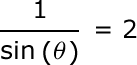#### Question 2 2. Solve the following equation for θ.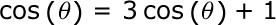#### Question 3 3. Solve the following equation for x.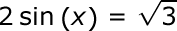#### Question 4 4. Solve for the value of x in the trigonometric equation below. (Use the domain -π/2 to π/2)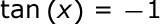#### Question 5 5. Solve the following trigonometric equation for x.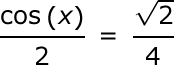#### Solving Trigonometric Equations: Homework Help Chapter Exam Instructions

Choose your answers to the questions and click 'Next' to see the next set of questions. You can skip questions if you would like and come back to them later with the yellow "Go To First Skipped Question" button. When you have completed the practice exam, a green submit button will appear. Click it to see your results. Good luck!

Support